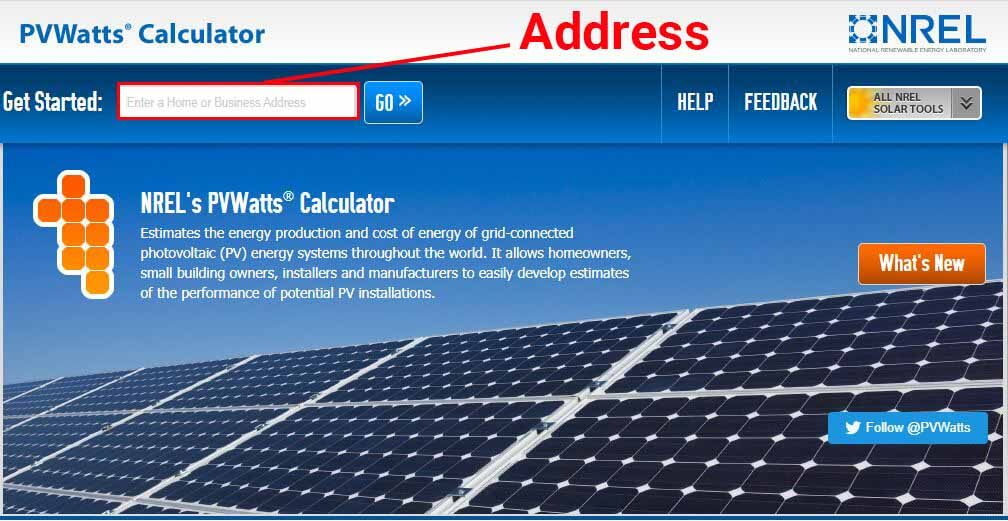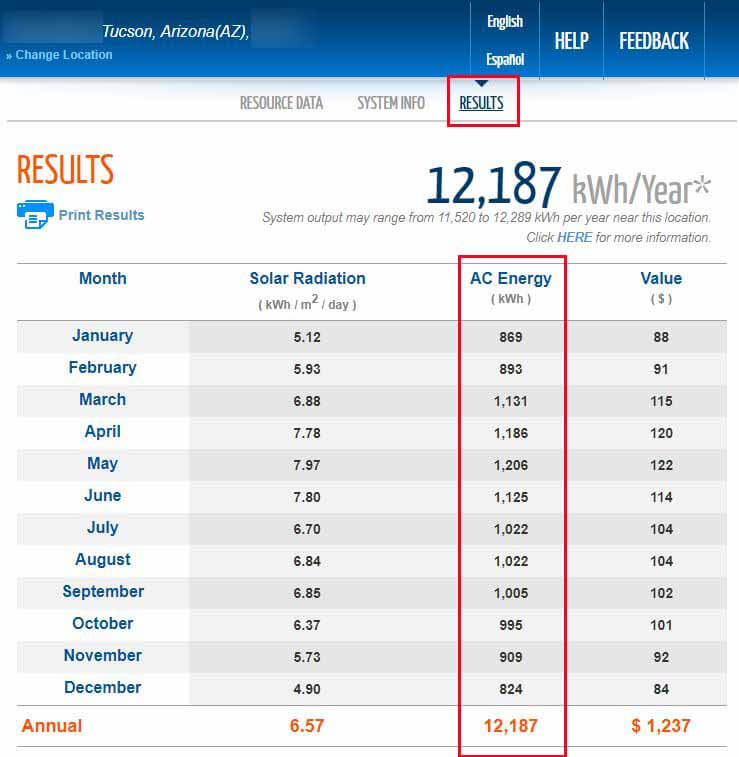# How much does a 7kw solar system produce?

A 7kW rating on a solar system means that the system is potentially capable of producing 7 kilowatts (7000 watts) of power at a given moment. But this amount of power production is not guaranteed and would require a certain amount of sunlight to happen.

In other words, a 7kW solar system can only produce 7kW of power if direct sunlight is available.

However, the amount of power that a solar system produces isn’t what matters the most. What really matters is the average amount of energy (kWh) that the system generates on a daily or monthly basis. And this amount of energy production will mainly depend on your location.

In this article, I will explain an easy way to determine the amount of energy that a 7kW solar system is expected to produce based on your location, and other details that describe the system.

## How much energy does a 7kw system produce?

Electrical Power and Electrical Energy are often confused. While power is instantaneous and is measured in kW (kiloWatts), energy is measured over time (hours, days, months) and its measurement unit is kWh (kilowatt-hours). So the question is, how many kWh does a 7kw solar system produce?

As a rule of thumb, a 7kW solar system will typically generate 28 to 40 kWh (kiloWatt-hours) of energy per day, which translates to 850 – 1200 kWh of energy per month. However, the average amount of energy that a 7kW solar system produces, will mainly depend on the location in which it’s installed.

To set your expectations more accurately, you can use the PVWatts Calculator, which is a free tool provided by The National Renewable Energy Laboratory (NREL).

Based on a few variables that describe your system, the PVWatts Calculator estimates the average amount of energy (monthly and yearly) that a solar system is expected to produce. These variables are:

• Location
• DC System Size (kW)
• Tilt Angle
• Azimuth

The first step is to go to the PVWatts Calculator and submit your address:When you submit your address, the next step is to go to the System Info section of the calculator and modify the default settings:In this section of the tool, you’ll see 6 variables that you can modify. For simplicity, I’ll focus on the variables that I’ve mentioned above.

DC System Size (kW):

The first input to modify is the size (power rating) of the system. Since we’re discussing 7kW systems, simply enter 7.

Tilt (deg):

This represents the angle at which your solar panels will be tilted. The tilt angle of a solar panel influences the amount of sunlight that it receives, and therefore, the amount of energy that the solar panel produces.

For example, in the winter when the sun is closer to the horizon, a steeper tilt angle will generally result in higher energy production.

If this is a roof-mounted system, you can click on the information “i” icon and find the equivalent of your Roof Pitch in degrees. For example, a 5/12 Roof Pitch translates to 22.6 degrees.

If it’s a ground-mounted (open rack) system, or you just don’t know what your Roof Pitch is, you can use an app such as Measure (for iOS) or Bubble Level (for Android) to determine the tilt angle.

Azimuth (deg):

Azimuth represents the direction that the solar panels are facing, and it also influences the amount of energy that the system produces. For example, if the solar panels are facing East, the Azimuth is 90 degrees. If the solar panels are facing South-East, the Azimuth is 135 degrees.

To determine the Azimuth, you can use compass apps such as Commander Compass Go (for iOS) or Azimuth Compass (for Android).

Once you’ve modified these inputs, you can go to the Results section of the calculator to check out the results.

To give you an example, I’ve submitted the following details to the calculator:

• DC System Size: 7kW.
• Tilt (deg): a 5/12 roof pitch, which equates to 22.6 degrees.
• Azimuth: I’ll assume the roof section is facing South, the Azimuth for South is 180 degrees.

In the Results section, the calculator provided the following table:Based on the details that I’ve provided to it, the PVWatts calculator has estimated that a 7kW solar system would generate 12187 kWh of energy every year.

The energy production fluctuates depending on the month. However, we could say that, in this location, with a 22.6 tilt angle, our South-facing 7kW system would – on average – produce 33.4 kWh/day or 1000 kWh/month.

## How many panels do I need for a 7kw solar system?

Residential solar panels can be rated at anywhere between 250 and 400 watts (0.25-0.4 kW) each. This means that you would need between 18 and 28 residential solar panels to create a 7kW solar system. The exact number of solar panels would depend on the individual power rating of the panels.

For example, if we use these 72cell LG NeON 2 solar panels, which are rated at 390 watts of power, we would need 18 of these panels to make up a 7kW system.

## How big is a 7kw solar array?

In general, a 7kW solar array would require 380 to 450 ft² (35-42 m²) of space. The amount of space that you need for a 7kW solar system would mainly depend on the efficiency of the solar panels used in the installation.

Learn more about the different types of residential solar panels and their dimensions here: How big is a solar panel?

If you have shading issues on your roof and suspect there’s not enough usable space for the 7kW solar system, please refer to this page: How many solar panels can you fit on your roof?